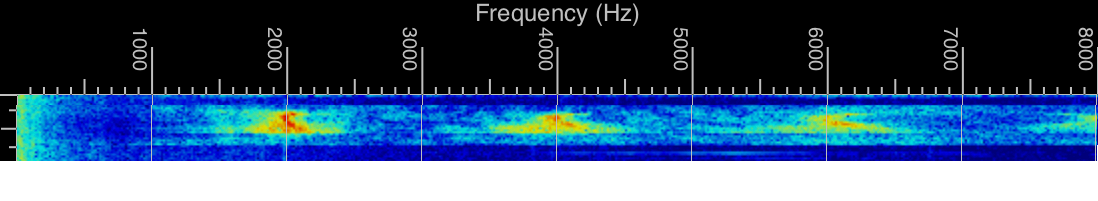# What is the pitch of squeaking chalk?

*chalk squeaks during lecture* “I did that intentionally, you know, to prevent people from falling asleep.”
–my freshman physics professor

At first glance, this problem might look excessively challenging for the physicist stranded on a desert island without samples of chalk. The microscopic mechanism of the chalk squeak is quite nontrivial, due to the velocity dependence of the kinetic friction coefficient between the chalk and the chalkboard. However, we find that the problem simplifies quickly.

The canonical example of the stick-slip mechanism is a mass on a horizontal spring. The mass is situated on a platform moving at a constant velocity (say, to the right). The block starts stationary; due to static friction, it is pulled to the right while remaining stationary relative to the platform. Once the spring overcomes static friction, the block is pulled leftwards. Now, the velocity dependence of the kinetic friction coefficient dominates the dynamics: the coefficient is small at very slow speeds; as the velocity increases, the coefficient rapidly increases to a peak, then it decays to a smaller value. Hence, when the spring overcomes static friction, the block rapidly slips leftwards. At some point, the block will return to rest relative to the platform; however, the distance of the slip depends on the peak and decay of the kinetic friction coefficient. Once the block sticks back on the platform, the cycle repeats, causing the stick-slip phenomenon.

Although the stick-slip behavior will cause an oscillation of a frequency highly dependent on the material parameters, the fact that the squeal is loud suggests a promising path forwards. The squeal occurs in the chalk itself, not the board. Hence, we can directly evaluate the resonant frequency of the chalk to recover the squeal frequency. This only depends on two parameters: the length of the chalk and the speed of sound in the chalk.

Before tackling sound in a solid, we recall the simpler case of a wave on a string. Since the speed of a propagating wave is not an extensive quantity in the length of the string, the string has only two relevant parameters: $$\mu$$ (mass per length) and $$T$$ (tension). By dimensional analysis (or the wave equation), the speed of the wave is $$c \sim \sqrt{T/\mu}$$. Scaling up to three dimensions, we require the analogue of tension: elastic modulus. The speed of sound in a solid is then $$c \sim \sqrt{M/\rho}$$, where $$M$$ is Young’s elastic modulus and $$\rho$$ is the density.

Microscopically, elastic modulus is like a spring constant normalized by the length and area of the spring (again, to prevent it from being an extensive quantity). Given a quadratic approximation of the interatomic potential, we can model bonds as springs of length $$l$$ and spring constant $$k$$. We know that the energy of the spring is $$E \sim \frac{1}{2}kl^2$$; since this is a bond, $$E$$ must correspond to the cohesive energy, i.e., the energy required to break the bond. Evaluating $$M=k/l$$ in terms of $$E$$, we recover the quantity \begin{aligned} c \sim \sqrt\frac{M}{\rho} \sim \sqrt\frac{2E}{\rho l^3}. \end{aligned} The cohesive energy varies from material to material, but we can estimate it to order of magnitude from the hydrogen atom. We know that the binding energy of hydrogen is $$\mathrm{Ry}=13.6$$ eV and occurs at the Bohr distance $$a_0 \sim 0.5~\mathrm{Å}$$. Due to the $$1/r$$ scaling of a Coulomb potential, the cohesive energy is simply scaled to give \begin{aligned} c \sim \sqrt\frac{2\,\mathrm{Ry}\,a_0}{\rho l^4}. \end{aligned} The lowest resonant frequency of the chalk will be its natural harmonic along the long axis of length $$L$$. Consequently, the fundamental frequency of wavelength $$\lambda = 2L$$ will be \begin{aligned} f \sim \frac{c}{2L} \sim \frac{1}{Ll^2}\sqrt\frac{\mathrm{Ry} \,a_0}{2\rho}. \end{aligned} If the chalk is a new piece of Crayola chalk ($$l~5~\mathrm{cm}$$, $$\rho=2~\mathrm{g/cm^3}$$), we obtain a frequency of \begin{aligned} f \sim \frac{340~\mathrm{kHz}}{\tilde{l}^2}, \end{aligned} where $$\tilde{l}$$ denotes the estimated bond length in angstroms. Due to the square dependence in $$\tilde{l}$$, our estimate is slightly sensitive to the choice of bond length. Given the strong dipole moment between Ca$$^{2+}$$ and CaCO$$_3^{2-}$$ in chalk (calcium carbonate), we assume that molecules of chalk are bonded by dipole-dipole interactions with $$l \sim 10~\mathrm{Å}$$. (In contrast, a hydrogen bond would have $$l \sim 3~\mathrm{Å}$$. For a street-fighting estimate of bond lengths, see our estimate for the age of the solar system.) Consequently, the resonant frequency of the chalk is approximately 3 kHz, or about three to four octaves above middle C.

## Experimental results

The day after completing this computation, I performed the necessary experiment in the Berkeley math department. A new piece of Crayola chalk and a standard blackboard produced the below image, obtained by screenshotting a spectrogram on Jamie Simon’s phone. The 2 kHz measurement is close to our prediction of 3 kHz; the error is likely due to our glib choice of bond lengths.Spectrogram of a new piece of Crayola chalk squeaking on a blackboard, showing a natural resonance frequency of 2 kHz and higher-order harmonics an octave, an octave-and-a-half, and two octaves higher.

## References

For the speed of sound, see Chapter 3 of .

 Goldreich, Peter, Sanjoy Mahajan, and Sterl Phinney. “Order-of-magnitude physics: Understanding the world with dimensional analysis, educated guesswork, and white lies.” University of Cambridge (1999).